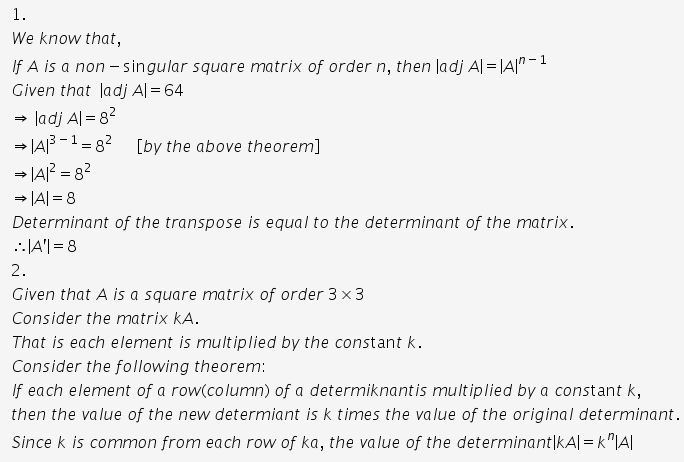# If A is a square matrix of order 3 such that |Adj A|=64, find|A'|

1. If A is a square matrix of order 3 such that |Adj A|=64, find|A’|.
2. If A is a square matrix of order 3x3, then find |kA|.